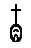## Thursday, June 20, 2019

### The symbol of deathAzrael, the angel of death Evelyn De Morgan (1855-1919)
For an educated classical Greek, the number 8 represented death. Why? Let’s see what this funeral assignment was based on.
1. Multiply by 8 the first 8 natural numbers.
2. Add the digits for each result.
3. If the total obtained has more than one digit, we add those digits again.

 Multiply Add digits 2nd addition 1×8=8 8 8 2×8=16 1+6=7 7 3×8=24 2+4=6 6 4×8=32 3+2=5 5 5×8=40 4+0=4 4 6×8=48 4+8=12 1+2=3 7×8=56 5+6=11 1+1=2 8×8=64 6+4=10 1+0=1

Observe that we obtain the sequence 8,7,6,5,4,3,2,1. For the Greeks, this succession starts at 8 and descends to die at 1. That is why number 8 represented death.
Remember that the Greeks did not have a zero, so the death of the succession of decreasing numbers took place at value 1.
Zero is a very special number, which has in principle two different meanings, although at bottom they are related:
• Number zero, the limit of many decreasing and increasing successions, located between the succession of positive numbers (1,2,3...) and the succession of negative numbers (-1, -2, -3...). This should be the real symbol of death.
• Positional zero, which in our numerical notation of base 10 tells us that a number is missing (i.e. there is a jump in the power series of 10). Thus, 107 means that to obtain this value we must add one hundred and seven units, skipping the tens.Egyptian zero
Number zero appears for the first time in history in the Papyrus Boulaq 18, of the XIII dynasty, of the Egyptian Middle Kingdom, around the year 1700 a.e.C. The symbol used is read –nfr–. But the Egyptians did not know how to take advantage of the zero.
In Babylon, the numbering system used base 60. Unfortunately, since they did not have a symbol for positional zero, the numbers 12=1×60+2= 62; 102=1×60×60+2=3602; 1002=1×60×60×60+2=216,002, were represented in exactly the same way. The Babylonians do not seem to have been much worried about this.Maya symbol for zero
The Maya possessed a symbol for positional zero, documented since the year 36 a.e.C. Unfortunately, their numbering system, in base 20, had an anomaly in the number of hundreds, which in the case of dates was 18×20=360 (to approximate the number of days in a year), while in other numbers was 20×20=400. This complicates the calculations, which on the other hand were used mostly in Astronomy.
The Greeks, as has been said, did not know the zero. Ptolemy uses in the Almagest a positional symbol equivalent to zero, but considers it as a simple annotation, not as a digit that can be treated like any other. Therefore, the use of his symbol did not spread.
Our positional notation, with a zero equivalent to any other digit, which can be operated, dates back from the Indian civilization, around the 6th century. To represent the absence of a digit, they used the Sanskrit word shunya, which means void. The Arabs, who adopted the Indian notation, transformed that word into sifr. When the notation was transmitted towards the tenth century from Al-Andalus to Western Europe with the name of Arabic numeration, this word became, on the one hand, zero, on the other cipher.

This book by Clifford Pickover contains many riddles and anecdotes related to mathematics.

The same post in Spanish
Thematic Thread on Mathematics: Previous Next
Manuel Alfonseca

1.Hola Manuel,
The anglophone world does not use the apostrophe ' to symbolise multiplication, but either x or * or a central dot, or in algebra simply nothing, so ab means a*b.

Peter

1.Thanks, Peter, I was aware of that. In fact, I've used the x symbol (not the letter x) and I can see it in my navigator. This must be an incompatibility in yours (perhaps it doesn't have the Symbols font).

2.I have replaced the multiplication symbol by an alternative one that I hope works in every navigator. Can you confirm that you can now see it?